1. /
2. CBSE
3. /
4. Class 09
5. /
6. Science
7. /
8. NCERT Solutions for Class...

# NCERT Solutions for Class 9 Science Atoms and Molecules part 1### myCBSEguide App

Download the app to get CBSE Sample Papers 2023-24, NCERT Solutions (Revised), Most Important Questions, Previous Year Question Bank, Mock Tests, and Detailed Notes.

NCERT solutions for Science Atoms and Molecules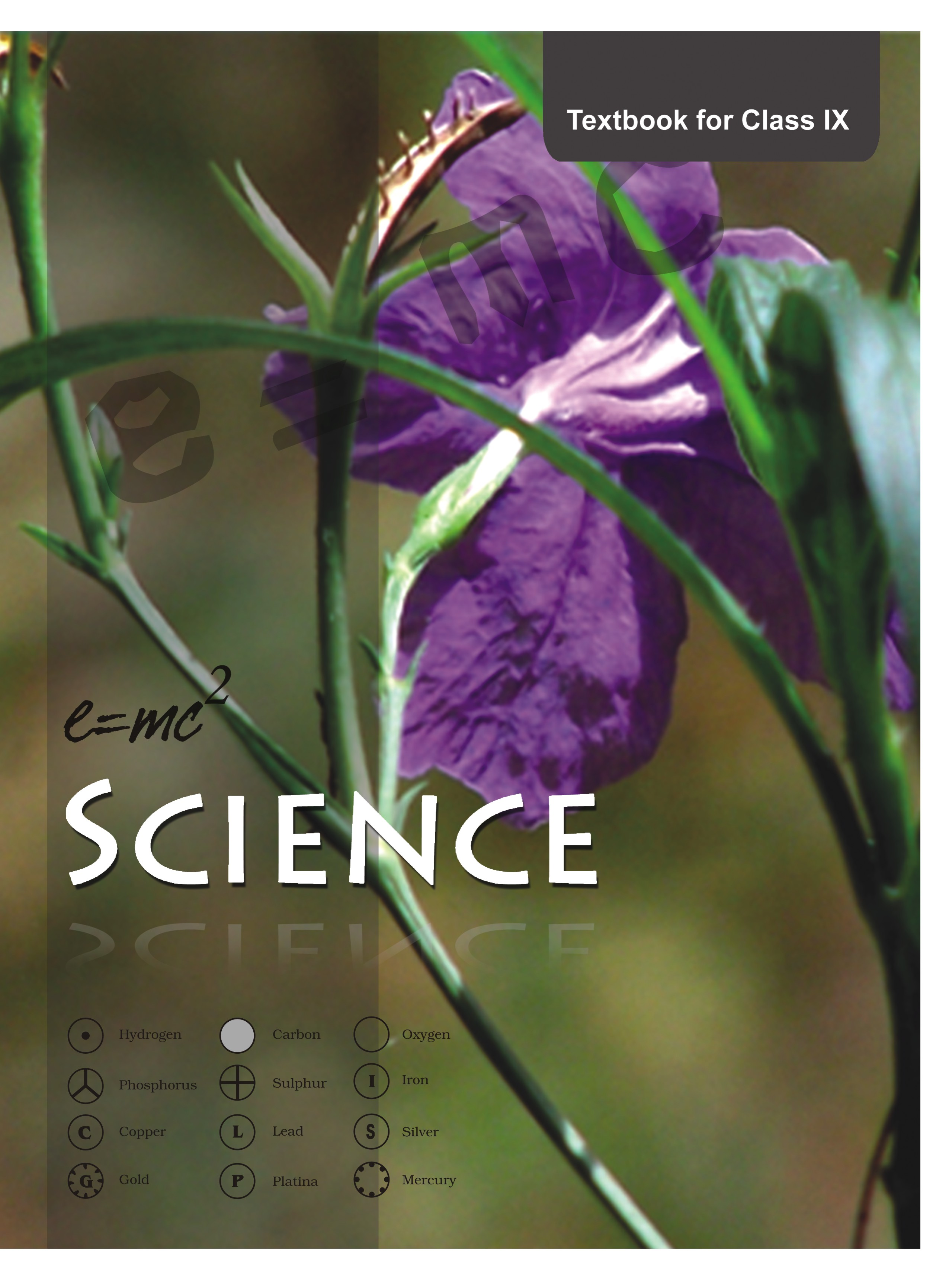## NCERT Class 9 Science Chapter wise Solutions

• 01 –  Matter in Our Surroundings
• 02 – Is Matter Around us Pure
• 03 – Atoms and Molecules
• 04 – Structure of the Atom
• 05 – The Fundamental Unit of Life
• 06 – Tissues
• 07 – Diversity in Living Organisms
• 08 – Motion
• 09 – Force and Laws of Motion
• 10 – Gravitation
• 11 – Word and Energy
• 12 – Sound
• 13 – Why Do We Fall Ill
• 14 – Natural Resources
• 15 – Improvement in Food Resources

## NCERT Solutions for Class 9 Science Atoms and Molecules part 1

###### 1. In a reaction, 5.3 g of sodium carbonate reacted with 6 g of ethanoic acid. The products were 2.2 g of carbon dioxide, 0.9 g water and 8.2 g of sodium ethanoate. Show that these observations are in agreement with the law of conservation of mass.

sodium carbonate + ethanoic acid → sodium ethanoate + carbon dioxide + water

Ans. According to law of conservation of mass:

mass of reactants = mass of products

Lets calculate and find out both results –

mass of reactants = mass of sodium carbonate +mass of ethanoic acid

= 5.3g + 6g

= 11.3g

mass of products = mass of sodium ethanoate + mass of carbon dioxide + mass of water

= 8.2g +2.2g + 0.9g = 11.3g

Hence it is proved that these observations are in agreement with the law of conservation of mass.

###### 2.Hydrogen and oxygen combine in the ratio of 1:8 by mass to form water. What mass of oxygen gas would be required to react completely with 3 g of hydrogen gas?

Ans. As per the given 1:8 ratio mass of oxygen gas required to react completely with 1g of hydrogen gas is 8g.

Therefore, mass of oxygen gas required to react completely with 3g of hydrogen gas will be =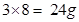###### 3.Which postulate of Dalton’s atomic theory is the result of the law of conservation of mass?

Ans. The postulate of Dalton’s atomic theory which is the result of the law of conservation of mass is mentioned as below:

Atoms are indivisible particles, which cannot be created or destroyed in a chemical reaction.

###### 4.Which postulate of Dalton’s atomic theory can explain the law of definite proportions?

Ans. The postulate of Dalton’s atomic theory which explains the law of definite proportions is “Atoms combine in the ratio of small whole numbers to form compounds and the relative number and kinds of atoms are constant in a given compound.”

NCERT Solutions for Class 9 Science Atoms and Molecules part 1

###### 1.Define the atomic mass unit.

Ans. According to the latest recommendations of International Union of Pure and Applied Chemistry (IUPAC) the atomic mass unit (amu) is abbreviated as u or unified mass.

For chemical calculations the atomic masses of elements are expressed by taking the atomic mass of one atom of an element as the standard mass. Like the atomic mass of carbon is taken as 12 units and each unit is called as 1 a.m.u i.e.

1 amu = 1/12 of atomic masses of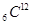.

NCERT Solutions for Class 9 Science Atoms and Molecules part 1

###### 2.Why is it not possible to see an atom with naked eyes?

Ans. An atom is an extremely minute particle and as such actual mass of an atom of hydrogen is considered to be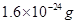. That is why it is not possible to see an atom with naked eyes.

(Page No. 39)

1.Write down the formulae of

(i)sodium oxide

(ii)aluminium chloride

(iii)sodium Sulphide

(iv)magnesium hydroxide

Ans.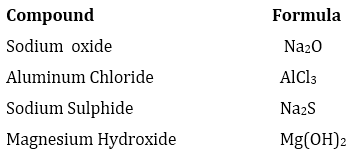###### 2.Write down the names of compounds represented by following formulae:

(i)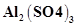(ii)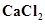(iii)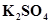(iv)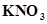(v)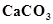.

Ans.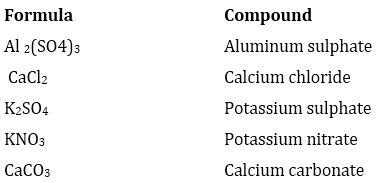###### 3.What is meant by the term chemical formula?

Ans. A chemical formula is the representation of elements present in a compound with the help of symbols and also the number of atoms of each element with those numbers only. for eg: A molecule of water (compound) contains 2 atoms of hydrogen and one atom of oxygen hence its chemical formula is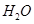.

###### 4.How many atoms are present in a

(i)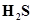molecule and

(ii)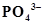ion?

Ans. (i) 2 atoms of hydrogen + 1 atom of sulphur = 3 atoms

(ii)1 atom of phosphorus + 4 atoms of oxygen = 5 atoms

NCERT Solutions for Class 9 Science Atoms and Molecules part 1

###### 1. Calculate the molecular masses of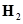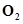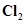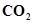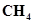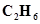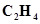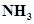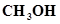Ans. Molecular mass of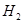= atomic mass of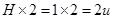.

Molecular mass of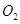= atomic mass of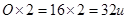.

Molecular mass of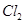= atomic mass of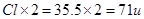.

Molecular mass of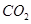= atomic mass of C + (atomic mass of O x 2)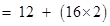= (12 + 32)

= 44 u

Molecular mass of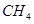= 12 + atomic mass of hydrogen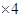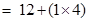= 12 + 4

= 16 u

Molecular mass of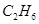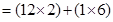= 24+6 = 30 u

Molecular mass of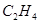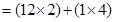= 24 + 4 = 28 u

Molecular mass of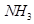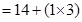= 14 + 3= 17 u

Molecular mass of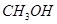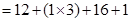= 12+3+16+1 = 32 u

NCERT Solutions for Class 9 Science Atoms and Molecules part 1

###### 2. Calculate the formula unit masses of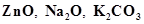, given atomic masses of Zn = 65 u, Na = 23 u, K = 39 u, C = 12 u, and O = 16 u.

Ans. Formula unit mass of:

i) ZnO = Atomic mass of Zn + atomic mass of O = (65 + 16) u = 81 u

ii)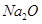= Atomic mass of Na + atomic mass of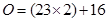= 46 + 16 = 62 u

iii)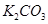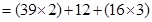= 78 + 12 + 48 = 138 u

NCERT Solutions for Class 9 Science Atoms and Molecules part 1

###### 1. If one mole of carbon atoms weighs 12 grams, what is the mass (in grams) of 1 atom of carbon?

Ans. Weight of one mole of carbon = atomic mass of carbon (1 atom of carbon) = 12 u

Therefore, one mole of carbon contains = 12 g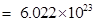atoms (Avogadro number)

so 1 atom of carbon = 12/ g

or, 12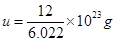1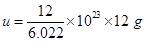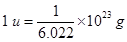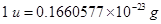or,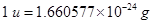NCERT Solutions for Class 9 Science Atoms and Molecules part 1

###### 2.Which has more number of atoms, 100 grams of sodium or 100 grams of iron (given, atomic mass of Na = 23 u, Fe = 56 u)?

Ans. We can find out the element with more number of atoms by calculating number of moles of each of them:

Number of moles of sodium in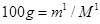= 100/23 = 4.34

Number of moles of iron in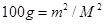= 100/56 = 1.79

Therefore, the number of atoms is more for sodium as compared to iron.

## NCERT Solutions for Class 9 Science

NCERT Solutions Class 9 Science PDF (Download) Free from myCBSEguide app and myCBSEguide website. Ncert solution class 9 Science includes text book solutions .NCERT Solutions for CBSE Class 9 Science have total 15 chapters. 9 Science NCERT Solutions in PDF for free Download on our website. Ncert Science class 9 solutions PDF and Science ncert class 9 PDF solutions with latest modifications and as per the latest CBSE syllabus are only available in myCBSEguide.

## CBSE app for Class 9

To download NCERT Solutions for class 9 Science, Computer Science, Home Science,Hindi ,English, Maths Social Science do check myCBSEguide app or website. myCBSEguide provides sample papers with solution, test papers for chapter-wise practice, NCERT solutions, NCERT Exemplar solutions, quick revision notes for ready reference, CBSE guess papers and CBSE important question papers. Sample Paper all are made available through the best app for CBSE students and myCBSEguide website.### Test Generator

Create question paper PDF and online tests with your own name & logo in minutes.### myCBSEguide

Question Bank, Mock Tests, Exam Papers, NCERT Solutions, Sample Papers, Notes